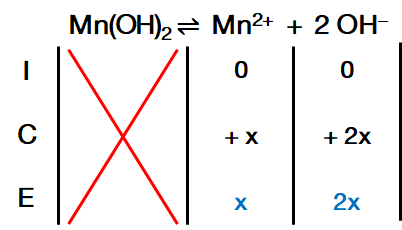# Problem: Calculate the mass of manganese hydroxide present in 1300 mL of a saturated manganese hydroxide solution. For Mn(OH)2, Ksp = 2.0 X 10 -13.

###### FREE Expert Solution

For Mn(OH)2:Ksp expression:

$\overline{){{\mathbf{K}}}_{{\mathbf{sp}}}{\mathbf{=}}\frac{\mathbf{products}}{\overline{)\mathbf{reactants}}}{\mathbf{=}}\mathbf{\left[}{\mathbf{Mn}}^{\mathbf{2}\mathbf{+}}\mathbf{\right]}{\mathbf{\left[}{\mathbf{OH}}^{\mathbf{-}}\mathbf{\right]}}^{{\mathbf{2}}}}$

Given: Ksp = 2.0 × 10–13

91% (85 ratings)###### Problem Details

Calculate the mass of manganese hydroxide present in 1300 mL of a saturated manganese hydroxide solution. For Mn(OH)2, Ksp = 2.0 X 10 -13.